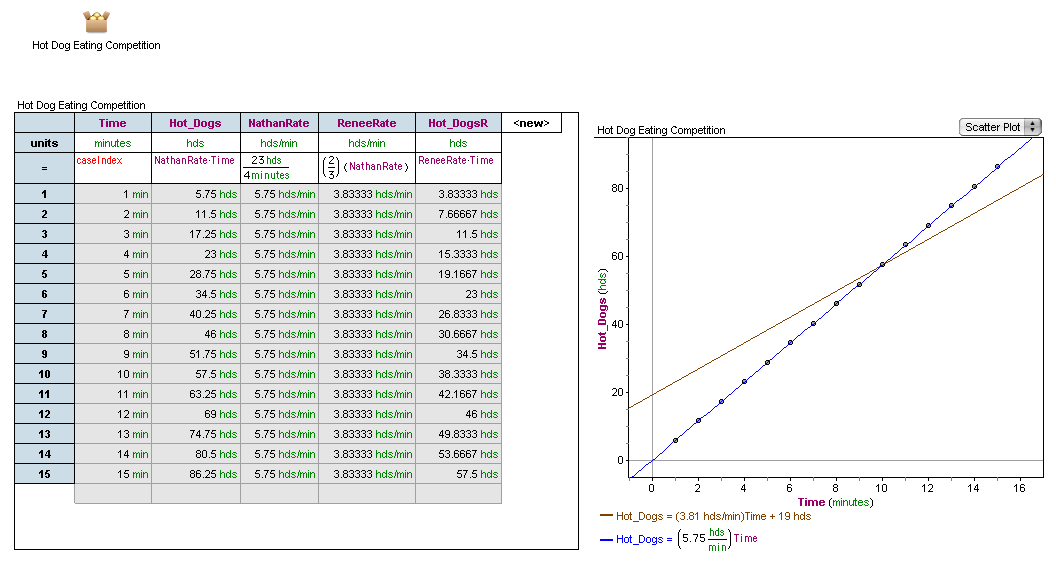# Hot Dog Eating Contest

Author:
hjelm

## Overview

Below is a photo of a fathom program that we used in class as an introduction to the program. We looked at a hot dog eating contest and compared the amount of hot dogs Nathan ate to the amount of time passed. From there, we found the rate of hot digs he ate per minute and graphed it. We then put in another example contestant who would eat 23 hot dogs in 6 minutes and compared their rate to Nathans and graphed it as well.Above we put in the correct formulas in each column that made sense for each category. This then gave us the information to graph the amount of hot dogs eaten to the time it took to eat. Also, we were told that another contestant can eat the amount of hot dogs that Nathan could eat, and that they can eat 23 hot dogs in 6 minutes. We could then use formulas to give us all the information we needed to graph another line on the same graph. From here, we could see that the overlap would occur at 19 hot dogs.

This relates to Grade 9 Patterns and Relations (Patterns) section of the curriculum specifically number 2. The students have to: - Describe the pattern found in a given graph. - Graph a given linear relation, including horizontal and vertical lines. - Match given equations of linear relations with their corresponding graphs. - Extend a given graph (extrapolate) to determine the value of an unknown element. - Interpolate the approximate value of one variable on a given graph, given the value of the other variable. - Extrapolate the approximate value of one variable from a given graph, given the value of the other variable. - Solve a given problem by graphing a linear relation and analyzing the graph.

## 20-3

This also relates to 20-1 Statistics specifically number 1. They have to drag down a graph and gather information from them. The students are required to: - Determine the possible graphs that can be used to represent a given data set, and explain the advantages and disadvantages of each. - Create, with and without technology, a graph to represent a given data set. - Describe the trends in the graph of a given data set. - Interpolate and extrapolate values from a given graph. - Explain, using examples, how the same graph can be used to justify more than one conclusion. - Explain, using examples, how different graphic representations of the same data set can be used to emphasize a point of view. - Solve a contextual problem that involves the interpretation of a graph.

## Sample Questions:

Grade 9: Teachers can give the students a graph and they can try to find a linear equation that best fits the line. This can be used in fathom by making a graph and getting the students to use a movable line that best fits the given points. Grade 11: Teachers can give the students data and the students can use technology to graph the data. This can be done using fathom. The teacher can make a data set and the students can graph it by dragging the appropriate data to the appropriate x and y values. From there, they can gather information about the graph.

## Conclusion

To conclude, I think that fathom is a great program for the 20-3 stream. There are so many different things that fathom can offer with regards to statistics. The program makes it so easy to navigate the different options and use it seamlessly. I really like fathom for a simple way to teach and a simple program for students to learn and navigate.